# Applications of Biot Savart law

## (3) Comparison between Coulomb�s laws and Biot Savart laws

• Both the electric and magnetic field depends inversely on square of distance between the source and field point .Both of them are long range forces
• Charge element dq producing electric field is a scalar whereas the current element Idl is a vector quantity having direction same as that of flow of current
• According to coulomb�s law ,the magnitude of electric field at any point P depends only on the distance of the charge element from any point P .According to Biot savart law ,the direction of magnetic field is perpendicular to the current element as well as to the line joining the current element to the point P
• Both electric field and magnetic field are proportional to the source strength namely charge and current element respectively. This linearity makes it simple to find the field due to more complicated distribution of charge and current by superposing those due to elementary changes and current elements

## (4) Applications of Biot Savart law

In this section we will now apply Biot-Savart law as studied in previous section to calculate field B in some important cases

i) Magnetic Field due to steady current in an infinitely long straight wire
• Consider a straight infinitely long wire carrying a steady current I
• We want to calculate magnetic field at a point P at a distance R from the wire as shown below in figure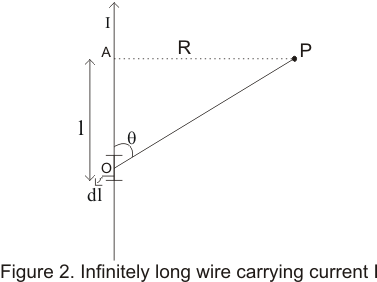• From Biot,-Savart law ,magnetic field dB due to small current element of the wire at point O at a distance |r|=r from point P is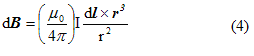• since current element Idl and vector r makes an angle θ with each other ,the magnitude of the product dlXr is dlrsinθ and is directed perpendicular to both dl and r vector as shown in the figure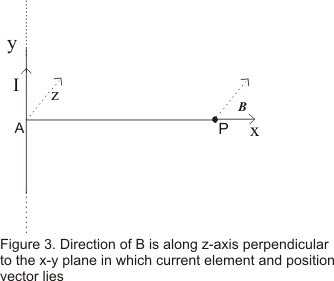• Since from our choice of co-ordinate, we found out that field B lies along z-axis therefore we can write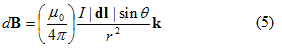where k is the unit vector along z-axis
• we will now express sinθ and r in terms of R which is fixed distance for any point in space and l which describes the position of current element on the infinitely long wire .From figure 1 we have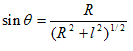and r=(R2 +l2)1/2
Putting these values in the equation (5) we find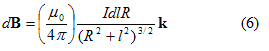• To find the field due to entire straight wire carrying wire ,we would have to integrate equation (6) B=∫dB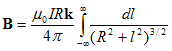• To evaluate the integral on the RHS substitute
l=RtanΦ and dl=Rsec2Φ dΦ
Therefore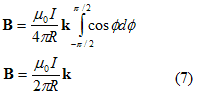• From equation (7) ,we noticed that
i) Magnetic field is proportional to the current I
ii) It is inversely proportional to the distance R
iii)Magnetic field is in the direction perpendicular to the straight wire and vector AP=R
• The magnetic line of force near a linear current carrying wire are concentric circles around the conductor in a plane perpendicular to the wire
• Hence the direction of field Bat point P at a distance R from wire, will be along the tangent drawn on a circle of radius R around the conductor as shown below in figure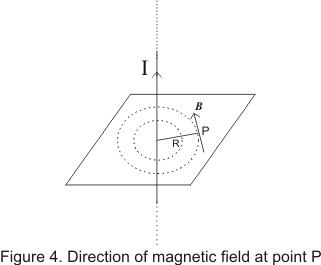Direction of B
• Direction of B can be found by right hand thumb rule i.e. grasp the wire with right hand ,the thumb pointing in direction of current ,the finger will curl around the wire in the direction of B
• The magnetic field lines are circular closed curve around the wire

Note to our visitors :-

Thanks for visiting our website.